Home / Expert Answers / Finance / on-1-january-205-franco-ltd-purchased-530-000-of-gentron-company-7-00-bonds-the-bonds-pay-se-pa575

# (Solved): On 1 January 205, Franco Ltd. purchased $530,000 of Gentron Company 7.00% bonds. The bonds pay se ...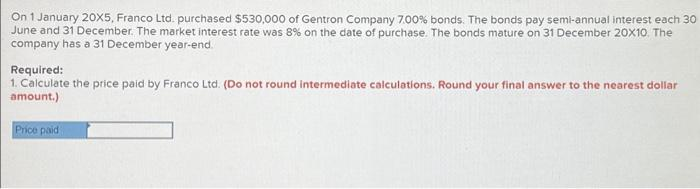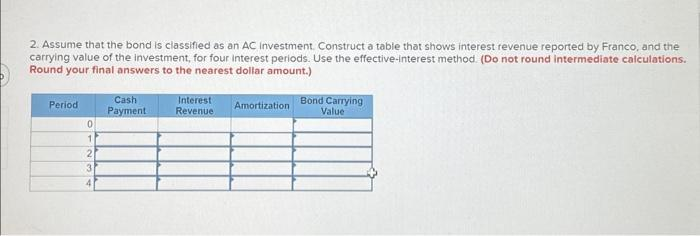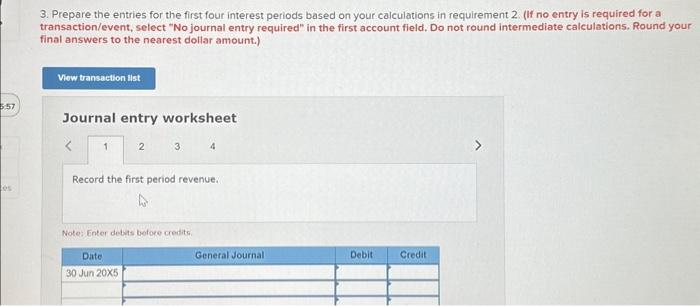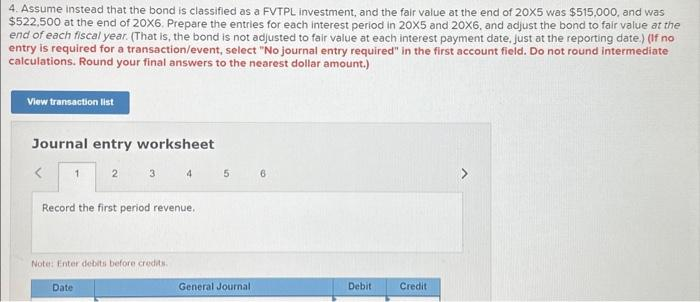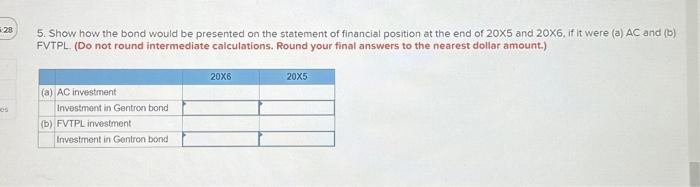On 1 January , Franco Ltd. purchased of Gentron Company bonds. The bonds pay semk-annual interest each 30 June and 31 December. The market interest rate was on the date of purchase. The bonds mature on 31 December . The company has a 31 December year-end Required: 1. Calculate the price paid by Franco Ltd. (Do not round intermediate calculations. Round your final answer to the nearest dollar amount.) 2. Assume that the bond is classified as an AC investment. Construct a table that shows interest revenue reported by Franco, and the carrying value of the investment, for four interest periods. Use the effective-interest method. (Do not round intermediate calculations. Round your final answers to the nearest dollar amount.) 3. Prepare the entries for the first four interest periods based on your calculations in requirement 2 . (If no entry is required for a transaction/event, select "No journal entry required" in the first account field. Do not round intermediate calculations. Round your final answers to the nearest dollar amount.) Journal entrv worksheet 4. Assume instead that the bond is classifled as a FVTPL investment, and the fair value at the end of was , and was at the end of . Prepare the entries for each interest period in and , and adjust the bond to fair value at the end of each fiscal year. (That is, the bond is not adjusted to fair value at each interest payment date, Just at the reporting date.) (If no entry is required for a transaction/event, select "No journal entry required" in the first account field. Do not round intermediate calculations. Round your final answers to the nearest dollar amount.) 5. Show how the bond would be presented on the statement of financial position at the end of and 20X6, if it were (a) AC and (b) FVTPL (Do not round intermediate calculations. Round your final answers to the nearest dollar amount.) We have an Answer from Expert ### Expert Answer 1. To calculate the price paid by Franco Ltd. for the Gentron Company bonds, we need to determine the present value of the bond's cash flows. Step 1: Calculate the present value of the bond's interest payments. The bond pays semi-annual interest, so there will be 2 payments per year for a total of 10 periods (5 years). The coupon payment is 7.00% of the face value, which is$530,000. Using the formula for the present value of an annuity, we can calculate the present value of the interest payments:

PV of Interest Payments = Coupon Payment × [1 - (1 + r)(-n)] / r Where: Coupon Payment = $530,000 × 7.00% / 2 =$18,550 r = Market interest rate / 2 = 8% / 2 = 4% (since it's a semi-annual rate) n = Number of periods = 10 PV of Interest Payments = $18,550 × [1 - (1 + 4%)(-10)] / 4% =$150,457.117

We have an Answer from Expert